# Valance Electron Theories and Orbital Overlap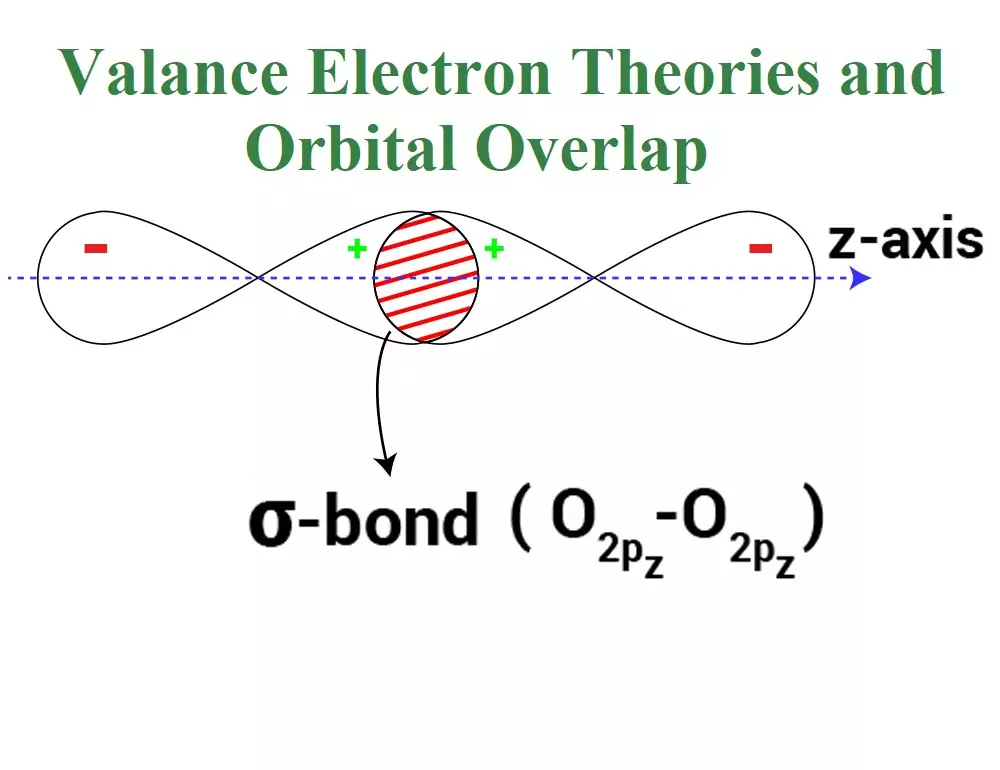Valance Electron Theories and Orbital Overlap

Valance Electron Theories and Orbital Overlap:

The valence shell electron pair repulsion (VSEPR) Theory:
This theory was proposed by Sidgwick and Powell and later modified by Nyholm and Gillespie. The important postulates of this theory are:
(i) The shape of a molecule depends upon the number of valence shell electron pairs (bonded or nonbonded) around the central atom.
(ii) Pairs of electrons in the valence shell repel one another since their electron clouds are negatively charged.
(iii) These pairs of electrons tend to occupy such positions in space that minimize repulsion and thus maximize the distance between them.
(iv) The valence shell is taken as a sphere with the electron pairs localizing on the spherical surface at maximum distance from one another.
(v) A multiple bond is treated as if it is a single electron pair and the two or three electron pairs of multiple bonds are treated as a single super pair.
(vi) Where two or more resonance structures can represent a molecule, the VSEPR model is applicable to any such structure.
(vii) The repulsive interaction of electron pairs decreases in the order: Lone pair (lp) – Lone pair (lp) > Lone pair (lp) – Bond pair (bp) > Bond pair (bp) –Bond pair (bp)

Shapes of the Molecules or Ions with Bond Pairs of electrons on the Central Atom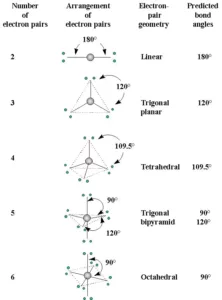Shapes of the Molecules or Ions with Lone Pairs and Bond Pairs of electrons on the Central Atom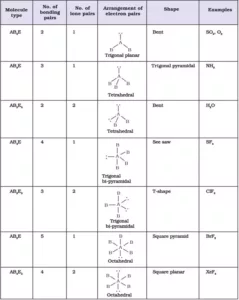Explanation of shapes of Molecules NH3 and H2O:
NH3: In ammonia, the central atom N has 5 valence electrons (7N – 2,5). Among these electrons, three are used for the formation of bonds with hydrogen atoms and the remaining 2 electrons stay as lone pairs. So, there are 4 VSEPs. Hence the expected shape of the molecule is tetrahedral. But due to the presence of lone pairs, the shape is distorted to trigonal bipyramidal, and the bond angle changes from 109028l to 1070.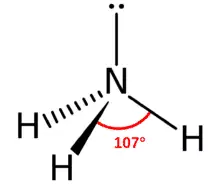H2O: In water, the central atom O has 6 valence electrons (8O – 2,6). Two of them are used for the formation of bonds with hydrogen atoms and the remaining 4 electrons stay as lone pairs. So there are 4 VSEPs. Hence the expected shape of the molecule is tetrahedral. But due to the presence of 2 lone pairs, the shape is distorted bent or angular or inverted v shape, and the bond angle changes from 109028l to 104.50.

Formal Charge: A formal charge (FC) is the charge assigned to an atom in a molecule. When determining the best Lewis Structure(or predominant resonance) for a molecule, the structure is chosen such that the formal charge on each of the atoms is as close to zero as possible.
Formula to Calculate Formal Charge: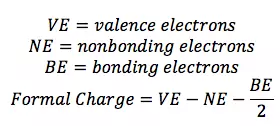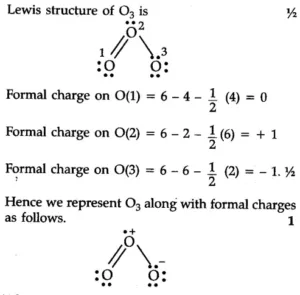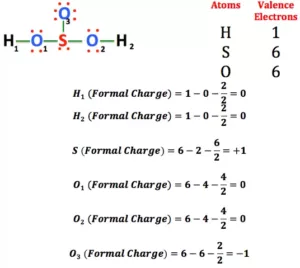Uses of Formal Charge:
1. The formal charge being a theoretical charge doesn’t indicate any real charge separation in the molecule.
2. Formal charges help in the selection of the lowest energy structure from a number of possible Lewis structures for a given species.
3. Knowledge of the lowest energy structure helps in predicting the major product of a reaction and also describes a lot of phenomena.
4. Generally, the lowest energy structure is the one with the smallest formal charges on the atoms and the most distributed charge.

Valence Bond Theory: This theory was introduced by Heitler and London and later developed by Linus Pauling in order to explain the shape of molecules theoretically. This theory can be described in the following points.
(i) A covalent bond is formed when the orbital of one atom is situated in such a way that it overlaps with the orbital of another atom, each of them containing one unpaired electron.
(ii) The atomic orbitals overlap and the overlapped region is occupied by both the electrons. These two electrons must have opposite spin.
(iii) As a result of this overlapping, there is a maximum electron density between the two atoms. A large part of the binding force of the covalent bond results from the attraction of these electrons by the nuclei of both atoms.
(iv) Atoms maintain their individuality. When the bond is formed, only valence electrons from each bonded atom are involved and the inner atomic orbitals of each atom remain undisturbed.
Consider 2 hydrogen atoms A and B with nucleus NA and NB and electrons eA and eB respectively. When the two atoms are at a large distance from each other, there is no further interaction between them. Due to this their potential energy is zero. When the two atoms start approaching each other, new attractive and repulsive forces begin to operate.
The attractive forces arise between the nucleus of one atom and the electron of another atom i.e., NA– eB, and NB– eA. Similarly, repulsive forces arise between electrons of two atoms i.e., eA – eB, and the nuclei of two atoms NA – NB. Attractive forces bring the two atoms close to each other whereas repulsive forces push them away.
The magnitude of the new attractive force is more than the new repulsive forces. So, the two atoms approach each other and potential energy decreases. At a particular stage, the net attractive force balances the net repulsive forces and the energy becomes minimum. At this stage, the hydrogen atoms are said to be bonded together to form a stable molecule.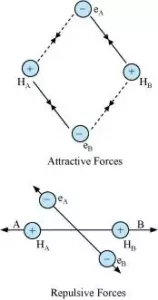Let us discuss another example of the formation of He2 molecule in which the number of attractive forces are less in comparison to the number of repulsive interactions so the He2 molecule does not exist.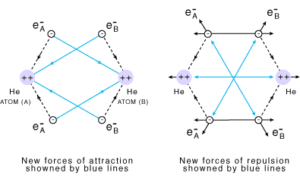Orbital Overlap Concept: The overlapping of the atomic orbital is the process of the partial interpenetration of atomic orbitals. The important characteristics of orbital overlapping are:
1. A covalent bond is formed by the overlapping of half-filled atomic orbitals present in the valence shell of atoms
2. The overlapping orbitals should contain an electron with opposite spin
3. Due to overlapping, the electrons get paired and a stable covalent bond is formed
4. The strength of a covalent bond depends on the extent of overlapping. The greater the extent of overlapping, the stronger will be the covalent bond formed

Types of Overlapping: There are two types of orbital overlapping.

Axial Overlapping or Sigma Bond: This type of covalent bond is formed by the end to end (hand-on) overlap of bonding orbitals along the internuclear axis. This is called head-on overlap or axial overlap. All single bonds are sigma bonds. A sigma bond can be formed in the following ways:
(i) s-s overlapping: Here the overlapping of two half-filled s-orbitals takes place along the internuclear axis.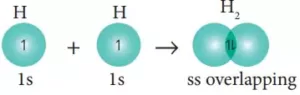(ii) s-p overlapping: Here the overlapping of half-filled s-orbitals and incomplete p-orbital take place along with the internuclear axis.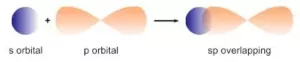(iii) p-p overlapping: Here two half-filled p- orbitals of two atoms overlap.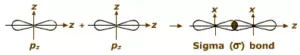pi(π ) bond: In the formation of π bond the atomic orbitals overlap in such a way that their axes remain parallel to each other and perpendicular to the internuclear axis or bond formed by sidewise overlapping of atomic orbitals called a pi bond. The orbitals formed due to sidewise overlapping consists of two charged clouds above and below the plane of the participating atoms.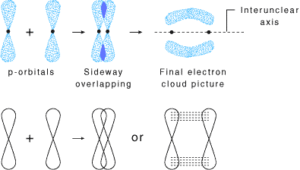A π bond is always present along with σ bonds. A double bond contains one σ bond and one π bond. A triple bond contains one sigma bond and two pi bonds.

A sigma bond is stronger than a pi bond. This is because the extent of overlapping is greater in a sigma bond.

Difference between Sigma and Pi Bond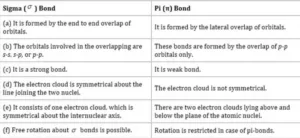Valance Electron Theories and Orbital Overlap